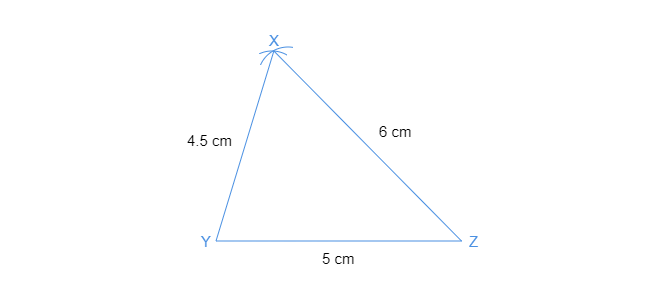# Construct $Ă˘Ë†â€ XYZ$ in which $XY=4.5\ cm,\ YZ=5\ cm$ and $ZX=6\ cm$.Thus, $\triangle XYZ$ is the required triangle.